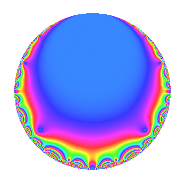Properties

 Label 34.2.aLevel 34 Weight 2 Character orbit a Rep. character $$\chi_{34}(1,\cdot)$$ Character field $$\Q$$ Dimension 1 Newforms 1 Sturm bound 9 Trace bound 0

Related objects

Defining parameters

 Level: $$N$$ = $$34 = 2 \cdot 17$$ Weight: $$k$$ = $$2$$ Character orbit: $$[\chi]$$ = 34.a (trivial) Character field: $$\Q$$ Newforms: $$1$$ Sturm bound: $$9$$ Trace bound: $$0$$

Dimensions

The following table gives the dimensions of various subspaces of $$M_{2}(\Gamma_0(34))$$.

Total New Old
Modular forms 6 1 5
Cusp forms 3 1 2
Eisenstein series 3 0 3

The following table gives the dimensions of the cuspidal new subspaces with specified eigenvalues for the Atkin-Lehner operators and the Fricke involution.

$$2$$$$17$$FrickeDim.
$$-$$$$+$$$$-$$$$1$$
Plus space$$+$$$$0$$
Minus space$$-$$$$1$$

Trace form

 $$q$$ $$\mathstrut +\mathstrut q^{2}$$ $$\mathstrut -\mathstrut 2q^{3}$$ $$\mathstrut +\mathstrut q^{4}$$ $$\mathstrut -\mathstrut 2q^{6}$$ $$\mathstrut -\mathstrut 4q^{7}$$ $$\mathstrut +\mathstrut q^{8}$$ $$\mathstrut +\mathstrut q^{9}$$ $$\mathstrut +\mathstrut O(q^{10})$$ $$q$$ $$\mathstrut +\mathstrut q^{2}$$ $$\mathstrut -\mathstrut 2q^{3}$$ $$\mathstrut +\mathstrut q^{4}$$ $$\mathstrut -\mathstrut 2q^{6}$$ $$\mathstrut -\mathstrut 4q^{7}$$ $$\mathstrut +\mathstrut q^{8}$$ $$\mathstrut +\mathstrut q^{9}$$ $$\mathstrut +\mathstrut 6q^{11}$$ $$\mathstrut -\mathstrut 2q^{12}$$ $$\mathstrut +\mathstrut 2q^{13}$$ $$\mathstrut -\mathstrut 4q^{14}$$ $$\mathstrut +\mathstrut q^{16}$$ $$\mathstrut -\mathstrut q^{17}$$ $$\mathstrut +\mathstrut q^{18}$$ $$\mathstrut -\mathstrut 4q^{19}$$ $$\mathstrut +\mathstrut 8q^{21}$$ $$\mathstrut +\mathstrut 6q^{22}$$ $$\mathstrut -\mathstrut 2q^{24}$$ $$\mathstrut -\mathstrut 5q^{25}$$ $$\mathstrut +\mathstrut 2q^{26}$$ $$\mathstrut +\mathstrut 4q^{27}$$ $$\mathstrut -\mathstrut 4q^{28}$$ $$\mathstrut -\mathstrut 4q^{31}$$ $$\mathstrut +\mathstrut q^{32}$$ $$\mathstrut -\mathstrut 12q^{33}$$ $$\mathstrut -\mathstrut q^{34}$$ $$\mathstrut +\mathstrut q^{36}$$ $$\mathstrut -\mathstrut 4q^{37}$$ $$\mathstrut -\mathstrut 4q^{38}$$ $$\mathstrut -\mathstrut 4q^{39}$$ $$\mathstrut +\mathstrut 6q^{41}$$ $$\mathstrut +\mathstrut 8q^{42}$$ $$\mathstrut +\mathstrut 8q^{43}$$ $$\mathstrut +\mathstrut 6q^{44}$$ $$\mathstrut -\mathstrut 2q^{48}$$ $$\mathstrut +\mathstrut 9q^{49}$$ $$\mathstrut -\mathstrut 5q^{50}$$ $$\mathstrut +\mathstrut 2q^{51}$$ $$\mathstrut +\mathstrut 2q^{52}$$ $$\mathstrut -\mathstrut 6q^{53}$$ $$\mathstrut +\mathstrut 4q^{54}$$ $$\mathstrut -\mathstrut 4q^{56}$$ $$\mathstrut +\mathstrut 8q^{57}$$ $$\mathstrut -\mathstrut 4q^{61}$$ $$\mathstrut -\mathstrut 4q^{62}$$ $$\mathstrut -\mathstrut 4q^{63}$$ $$\mathstrut +\mathstrut q^{64}$$ $$\mathstrut -\mathstrut 12q^{66}$$ $$\mathstrut +\mathstrut 8q^{67}$$ $$\mathstrut -\mathstrut q^{68}$$ $$\mathstrut +\mathstrut q^{72}$$ $$\mathstrut +\mathstrut 2q^{73}$$ $$\mathstrut -\mathstrut 4q^{74}$$ $$\mathstrut +\mathstrut 10q^{75}$$ $$\mathstrut -\mathstrut 4q^{76}$$ $$\mathstrut -\mathstrut 24q^{77}$$ $$\mathstrut -\mathstrut 4q^{78}$$ $$\mathstrut +\mathstrut 8q^{79}$$ $$\mathstrut -\mathstrut 11q^{81}$$ $$\mathstrut +\mathstrut 6q^{82}$$ $$\mathstrut +\mathstrut 8q^{84}$$ $$\mathstrut +\mathstrut 8q^{86}$$ $$\mathstrut +\mathstrut 6q^{88}$$ $$\mathstrut -\mathstrut 6q^{89}$$ $$\mathstrut -\mathstrut 8q^{91}$$ $$\mathstrut +\mathstrut 8q^{93}$$ $$\mathstrut -\mathstrut 2q^{96}$$ $$\mathstrut +\mathstrut 14q^{97}$$ $$\mathstrut +\mathstrut 9q^{98}$$ $$\mathstrut +\mathstrut 6q^{99}$$ $$\mathstrut +\mathstrut O(q^{100})$$

Decomposition of $$S_{2}^{\mathrm{new}}(\Gamma_0(34))$$ into irreducible Hecke orbits

Label Dim. $$A$$ Field CM Traces A-L signs $q$-expansion
$$a_2$$ $$a_3$$ $$a_5$$ $$a_7$$ 2 17
34.2.a.a $$1$$ $$0.271$$ $$\Q$$ None $$1$$ $$-2$$ $$0$$ $$-4$$ $$-$$ $$+$$ $$q+q^{2}-2q^{3}+q^{4}-2q^{6}-4q^{7}+q^{8}+\cdots$$

Decomposition of $$S_{2}^{\mathrm{old}}(\Gamma_0(34))$$ into lower level spaces

$$S_{2}^{\mathrm{old}}(\Gamma_0(34)) \cong$$ $$S_{2}^{\mathrm{new}}(\Gamma_0(17))$$$$^{\oplus 2}$$